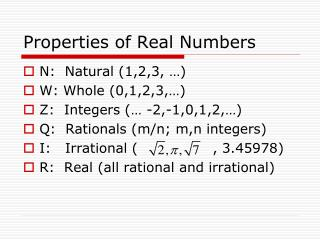DownloadDownload PresentationProperties of Real Numbers

# Properties of Real Numbers

Download Presentation## Properties of Real Numbers

- - - - - - - - - - - - - - - - - - - - - - - - - - - E N D - - - - - - - - - - - - - - - - - - - - - - - - - - -
##### Presentation Transcript

1. Properties of Real Numbers • N: Natural (1,2,3, …) • W: Whole (0,1,2,3,…) • Z: Integers (… -2,-1,0,1,2,…) • Q: Rationals (m/n; m,n integers) • I: Irrational ( , 3.45978) • R: Real (all rational and irrational)

2. Real Numbers, R IMAGINARY I Q Z W N

3. Properties of Real Numbers

4. Properties of Real Numbers • Distributive property • For all real numbers a, b, and ca(b+c) = ab + ac and (b+c)a = ba + ca

5. Solving Equations; 5 Properties of Equality

6. Solving Equations; 5 Properties of Equality

7. Applications of Equations Problem Solving Plan • Explore the Problem • Plan the solution • Solve the problem • Examine the solution

8. Absolute Value Equations Absolute value: Distance from zero For any real number a: If , then If , then